# Add Column to Pandas DataFrame with a Default Value

• Last Updated : 29 Aug, 2020

The three ways to add a column to Pandas DataFrame with Default Value.

• Using pandas.DataFrame.assign(**kwargs)
• Using [] operator
• Using pandas.DataFrame.insert()

## Using Pandas.DataFrame.assign(**kwargs)

It Assigns new columns to a DataFrame and returns a new object with all existing columns to new ones. Existing columns that are re-assigned will be overwritten.

Syntax: Pandas.DataFrame.assign(**kwargs)

Parameters : **kwargsdict of {str: callable or Series}

Returns : DataFrame

Let’s understand with examples:

First, create a simple DataFrame.

## Python3

 `# importing pandas as pd``import` `pandas as pd`` ` `# creating the dataframe``df ``=` `pd.DataFrame({``"Name"``: [``'Anurag'``, ``'Manjeet'``, ``'Shubham'``,``                            ``'Saurabh'``, ``'Ujjawal'``],`` ` `                   ``"Address"``: [``'Patna'``, ``'Delhi'``, ``'Coimbatore'``,``                               ``'Greater noida'``, ``'Patna'``],`` ` `                   ``"ID"``: [``20123``, ``20124``, ``20145``, ``20146``, ``20147``],`` ` `                   ``"Sell"``: [``140000``, ``300000``, ``600000``, ``200000``, ``600000``]})`` ` `print``(``"Original DataFrame :"``)``display(df)`

Output:## Python3

 `new_df ``=` `df.assign(profit``=``[``40000``, ``20000``, ``30000``, ``60000``, ``200000``])``new_df`

Output: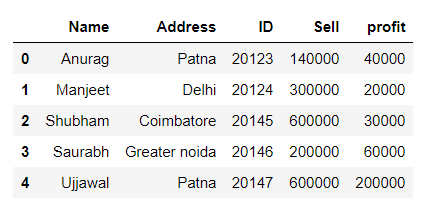Add a new column with Default Value:

## Python3

 `new_df ``=` `df.assign(profit``=``'NAN'``)``new_df`

Output: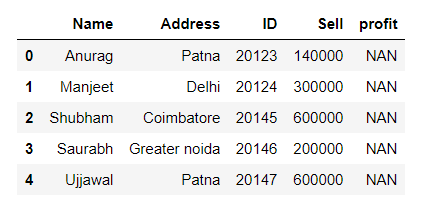## Using [] operator to add a new column

We can use DataFrame indexing to create a new column in DataFrame and set it to default values.

Syntax:

```df[col_name]=value
```

Let’s understand with an example:

## Python3

 `# importing pandas as pd``import` `pandas as pd`` ` `# creating the dataframe``df ``=` `pd.DataFrame({``"Name"``: [``'Anurag'``, ``'Manjeet'``, ``'Shubham'``,``                            ``'Saurabh'``, ``'Ujjawal'``],``                    ` `                   ``"Address"``: [``'Patna'``, ``'Delhi'``, ``'Coimbatore'``, ``                               ``'Greater noida'``, ``'Patna'``],``                    ` `                   ``"ID"``: [``20123``, ``20124``, ``20145``, ``20146``, ``20147``],``                    ` `                   ``"Sell"``: [``140000``, ``300000``, ``600000``, ``200000``, ``600000``]})`` ` `print``(``"Original DataFrame :"``)``display(df)`

Output:## Python3

 `df[``'loss'``] ``=` `[``40000``, ``20000``, ``30000``, ``60000``, ``200000``]``df`

Output: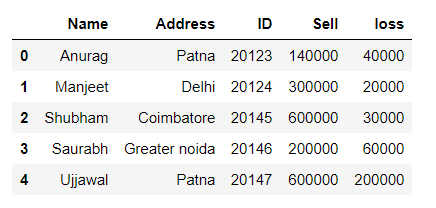Add a new column with default values:

## Python3

 `df[``'loss'``] ``=` `'NAN'``df`

Output: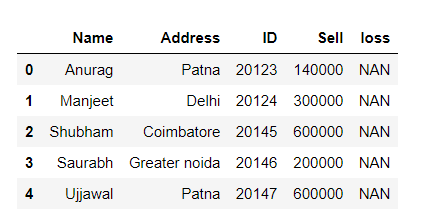## Using pandas.DataFrame.insert()

Add new column into DataFrame at specified location.

Syntax: DataFrame.insert(loc, column, value, allow_duplicates=False)

Parameters

loc : int Insertion index. Must verify 0 <= loc <= len(columns).

column : str, number, or hashable object Label of the inserted column.

value : int, Series, or array-like

allow_duplicates : bool, optional

Let’s understand with examples:

## Python3

 `# importing pandas as pd``import` `pandas as pd`` ` `# creating the dataframe``df ``=` `pd.DataFrame({``"Name"``: [``'Anurag'``, ``'Manjeet'``, ``'Shubham'``,``                            ``'Saurabh'``, ``'Ujjawal'``],``                    ` `                   ``"Address"``: [``'Patna'``, ``'Delhi'``, ``'Coimbatore'``, ``                               ``'Greater noida'``, ``'Patna'``],``                    ` `                   ``"ID"``: [``20123``, ``20124``, ``20145``, ``20146``, ``20147``],``                    ` `                   ``"Sell"``: [``140000``, ``300000``, ``600000``, ``200000``, ``600000``]})`` ` `print``(``"Original DataFrame :"``)``display(df)`

Output:Add a new column with default value:

## Python3

 `df.insert(``2``, ``"expenditure"``, ``4500``, allow_duplicates``=``False``)``df`

Output: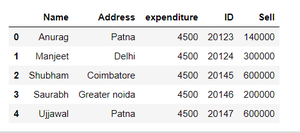My Personal Notes arrow_drop_up